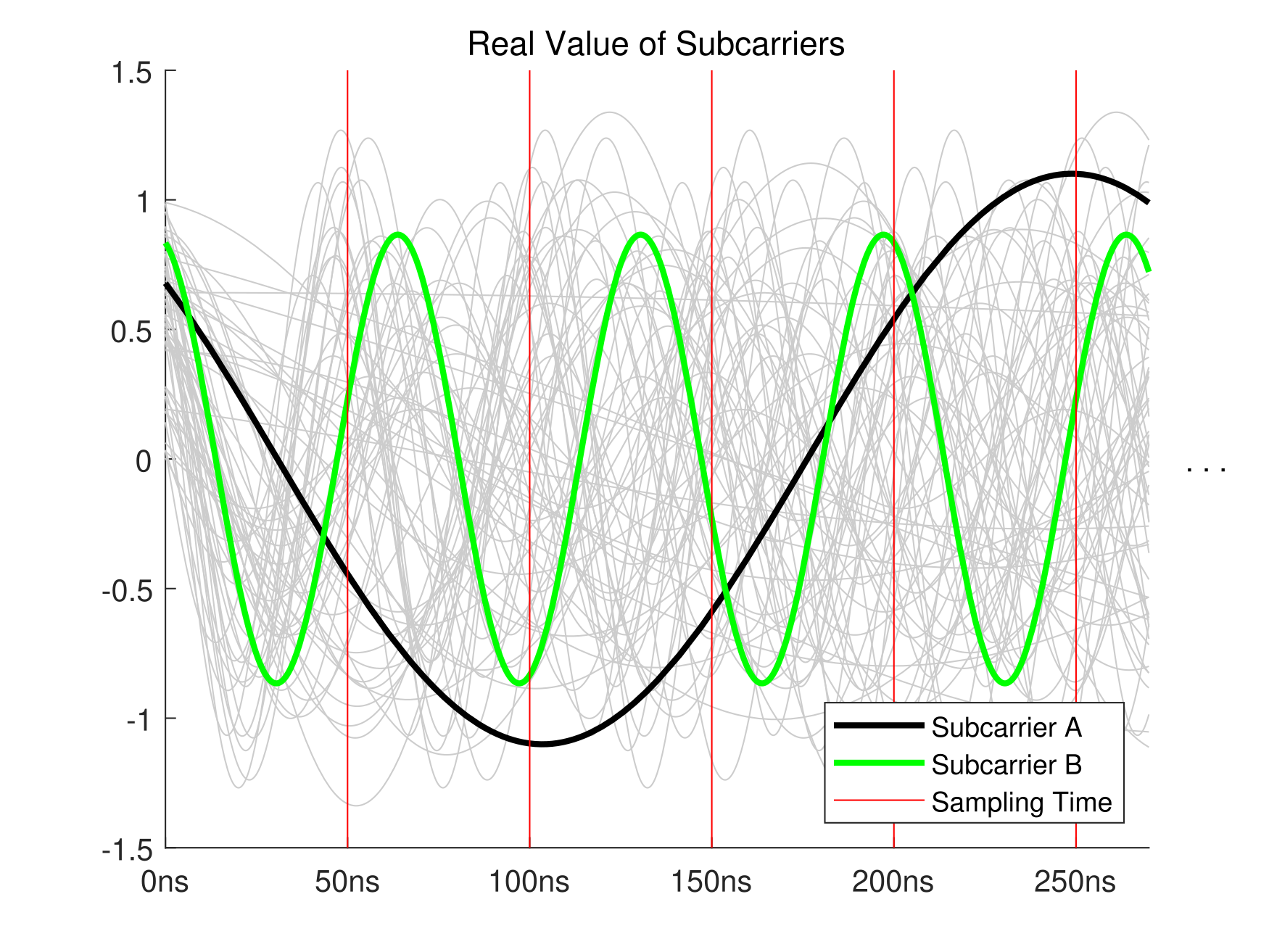블로그
글 개요
무선 네트워크/기본 개념 1개 있어요.# Where is the orthogonality in OFDM?

2020년 02월 04일 20:25무선 네트워크/기본 개념댓글 수
Mathematical Concept of "Orthogonality" Generally orthogonality means that the inner product of two vector is zero. There's not only a concept of the inner product of vectors, but the inner product of functions, and this can be expressed as a definite integral. \left\lbrace \begin{array}{ccc}=\int_a^b f(x)g(x)~dx & & \textrm{Inner}\;\textrm{Prod.}\;\textrm{of}\;\textrm{Real}\;\textrm{Fn.}\\=\i..
가장 위로